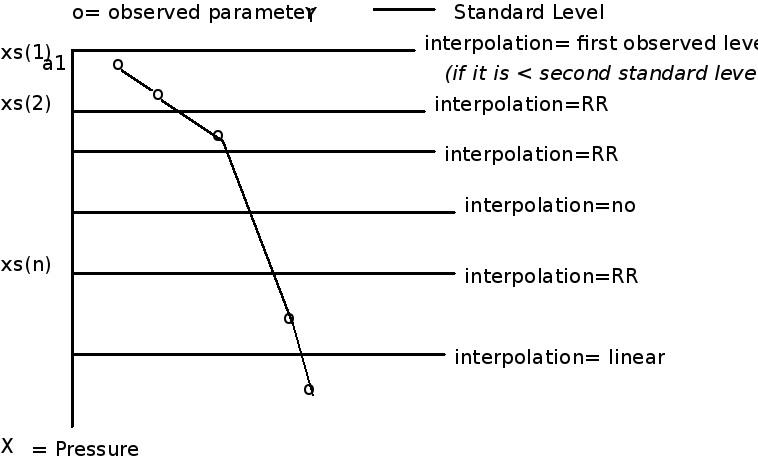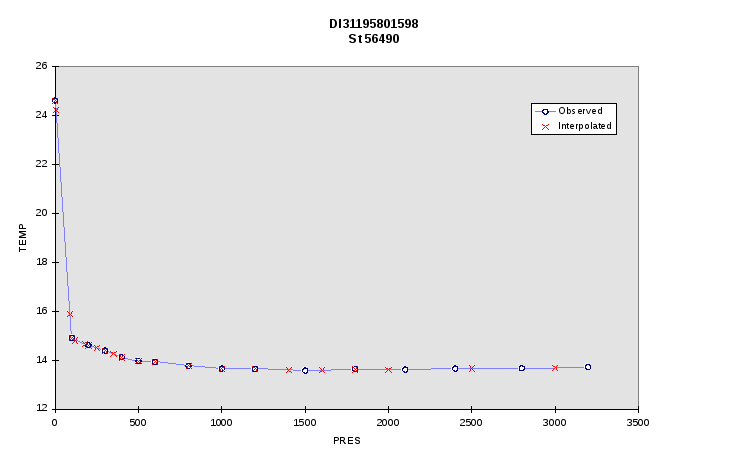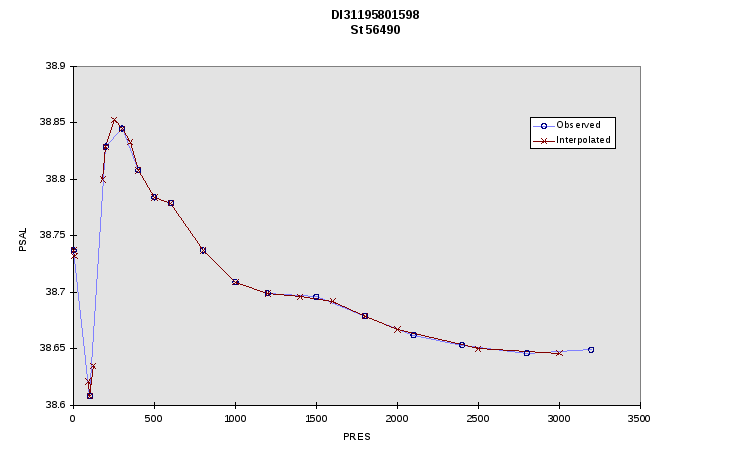# Direction de la Technologie Marine et des Systèmes d'Informations

 Page 10/10 Date 20.04.2018 Size 0.73 Mb.

#### Interpolation/Extrapolation

At levels where a mean value exists, but a standard deviation cannot be estimated it is necessary to::

• interpolate values where values exist above and below. The QC test was performed on the interpolated values. If an interpolated value was > max, then the interpolated value was replaced by the max value and if an interpolated value was < min, then the interpolated value was replaced by the min value

• last value when values only exist above it the QC test was performed on the extrapolated values

• first non default value for the extrapolation at the surface.

For all the extrapolated values, the minimum value of the QC envelope was taken, if the QC test did not succeed.

The averaged climatology can be accessed from the web site (www.ifremer.fr/medar/quality.htm)
The 4 files (annual, seasonal) are organised by vertical profiles in an auto descriptive ASCII format.
Example:
Mediterranean seasonal MEDATLAS climatology (release 1) per 1 degree square (0-800m)

Parameter: Temperature (Celsius.Degrees)

Parameter: Salinity (P.S.U.)

Period: Winter (months:01,02,03)

Latitude: +31.500000 Longitude: +32.500000

Mean value Standard deviation per parameter and immersion;

IMMER; TEMPERATURE;; SALINITY;;

0; 17.41; 2.00; 38.94; 0.13;

5; 17.37; 2.00; 38.92; 0.10;

10; 17.32; 2.00; 38.91; 0.10;

20; 17.30; 2.00; 38.96; 0.08;

30; 17.25; 2.00; 38.96; 0.07;

40; 17.20; 2.00; 38.97; 0.08;

50; 17.37; 2.00; 39.02; 0.08;

60; 17.27; 1.00; 38.94; 0.08;

80; 17.07; 0.90; 38.92; 0.08;

100; 16.90; 0.84; 38.94; 0.08;

120; 16.75; 0.76; 38.94; 0.08;

160; 16.29; 0.56; 38.98; 0.08;

200; 15.86; 0.41; 38.99; 0.08;

250; 15.40; 0.38; 38.98; 0.08;

300; 14.94; 0.31; 38.96; 0.08;

400; 14.23; 0.17; 38.92; 0.08;

500; 13.91; 0.04; 38.87; 0.08;

600; 99.99; 99.99; 99.99; 99.99;

800; 99.99; 99.99; 99.99; 99.99;

1. ## Test of the Redfield Ratio

An additional test is the Redfield ratio : the ratio of the oxygen, nitrate and alkalinity (carbonates) concentration over the phosphate concentration has been estimated respectively to 172, 16 and 122 by Takahashi & al. (13) in the Atlantic and Indian Ocean. New studies are made in the frame of MEDAR and CYCLOPS and these values will be adjusted for the Mediterranean in a near future.
These estimate allow a visual check on the corresponding nutrients, by checking the dispersion around the reference curve.
1. ## Standard level interpolation

The observed parameters were interpolated to the same standard levels as used in the climatological statistics .

Only data points with no outlier at all (Q flag=1) are used in the interpolation calculation.
1. ### Method and algorithms

The Reiniger & Ross (1968, here below referred as RR (11)) weighted parabolic interpolation is recommended by IOC and ICES and is widely used in the scientific community, in particular by Levitus at the WDC-A. The value of the correction made on the linear interpolation is a function of the difference between the linearly interpolated value and the two values extrapolated from the above and below levels.

The computation requires a profile of at least 4 levels. The authors extend the method for only 3 data points for the top and bottom of the profile, but the method is then not safe. In this case linear interpolation.was used.
In the case of no observed values between the standard level and the consecutive levels below and above, no interpolation is made.The RR linear reference function and the weights of the parabolic function depend on variable exponents. We took the same exponent values as RR, but these values may be adjusted later.
All the interpolated parameters are initialised with the default value at the standard levels.
1. ### Choice of the Mediterranean standard levels

For MEDATLAS 1997, the following 28 vertical levels have been processed:
0, 5, 10, 20, 30, 40, 50, 60, 80, 100, 120, 160, 200, 250, 300, 400, 500, 600, 800, 1000, 1200, 1400, 1600, 1800, 2000, 2500, 3000, et 4000 m
1. ### RR parabolic interpolation

The interpolated value of y (temperature or salinity) is a weighted mean of the two parabolic interpolations that use respectively the 3 superior data points and the 3 inferior data points.
The weights are a function of the difference between the parabolic interpolation and a reference function. This reference function is a combination of the linear interpolation and extrapolations using the 4 data points.
When none of the interpolating conditions are found, the parameter keeps the default values.
No control is made on the flags, only on the default values.
1. #### Conditions for computation

x=xs(n) y=ys(n)

a(1) < a(2) < x < a(3) < a(4)
First a check is made that the layers above and below are not both empty:
if a(2) < x(n-1) and a(3) > x(n+1) then ys(n) = default value

1. #### Algorithm:

dimension a(4),b(4)

the adjustable exponents are given the values used by RR

rm=2.

rn=1.
compute the parabolic interpolations

y1=fparb(x,a(1),a(2),a(3),b(1),b(2),b(3))

y2=fparb(x,a(2),a(3),a(4),b(2),b(3),b(4))
compute the reference function

yr=fref(x,a,b,rm)

compute the differences

d1=abs(yr-y1)**rn

d2=abs(yr-y2)**rn
compute the weights

r1=d1/(d1+d2)

r2=d2/(d1+d2)
compute the weihted mean

y=r1*y2+r2*y1

1. #### linear interpolation

function flin(x, a,b)

dimension a(2),b(2)

flin=b(1) + (x-a(1))*(b(2)-b(1))/(a(2)-a(1))

1. #### reference linear function

function fref(x,a,b,rm)

dimension a(4),b(4)

real x,a,b

if a(1) < a(2) < x < a(3) < a(4) and rm> 1. (else error)

then

y12=flin(x,a(1),a(2),b(1),b(2))

y23=flin(x,a(2),a(3),b(2),b(3))

y34=flin(x,a(3),a(4),b(3),b(4))
d123=(y12-y23)**rm

d234=(y23-y34)**rm

fref=0.5*(y23+(d234*y12 + d123*y34)/(d234+d123))

1. #### parabolic interpolation between 3 points

function fparab(x,a,b)

dimension a(3),b(3)

dimension gamma(3)

gamma(1)=(x-a(2))*(x-a(3)) / ((a(1)-a(2))*(a(1)-a(3)))

gamma(2)=(x-a(3))*(x-a(1)) / ((a(2)-a(3))*(a(2)-a(1)))

gamma(3)=(x-a(1))*(x-a(2)) / ((a(3)-a(1))*(a(3)-a(2)))
yp=gamma(1)*b(1)+gamma(2)*b(2)+gamma(3)*b(3)

fparab=yp

1. ### Top and bottom of the profile

1. #### First standard level xs(1)

ys(1) = fist observed parameter if the first observed level a(1) < xs(2)

else ys(1)=default value

1. #### Next standard level when there is only one observed level above

If there exists at least one point below the level, then the standard level is computed by using linear interpolation:

ys=flin(xs,a(1),a(2),b(1),b(2)) and flag=5 unless the standard level is an observed level
a(1), b(1) being the observed data point above the standard level and (a(2), b(2)) the observed data point below.

1. #### Last standard level at the bottom of the profile

When there is only one point below the level, the same linear interpolation is made.
1. ### Tests and Results

The software has been tested for the following:

• Input/output

- no change in the number of stations within the files

- output format

- not change in the location and date

- top and bottom of the profiles

- stations with low number of data points

- empty intermediate levels

• Control of the interpolated values

The computed interpolated values have been controlled at SISMER and a benchmark set of data sent to ICES who kindly gave assistance to detect the errors.

As an example, the processing of a station from the ATLANTIS 1958 in the deep Ionian Sea is plotted here below.## Références

(1) Intergovernmental Oceanographic Commission of UNESCO, 1998. MEDAR/MEDATLAS Meeting, Istanbul, Turkey, 21-23 May 1997 Summary Report, IOC/INF-1084.

(2) CEC/DG XII, MAST and IOC/IODE, 1993. Manual of Quality Control Procedures for validation of Oceanographic Data. UNESCO Manual and Guides N° 26, 436 P.

(3) Formatting Guidelines for Oceanographic Data Exchange. IOC/IODE/GETADE Report. IOC/ICES, Report.

(4) NOAA/NGDC, 1993. ETOPO5. 5-minute gridded elevation/bathymetry for the world. Global Relief Data. CDROM.

(5) JONES M.T., BODC, 1994. General Bathymetric chart of the Ocean, the GEBCO digital Atlas, CDROM.

(6) MEDATLAS Group. MEDATLAS 1997 Database and climatological atlas of temperature and salinity. 3 Cdroms, IFREMER Ed.

(7) BRASSEUR P., SCHOENAUEN R.. Seasonal Data Fields for the Mediterranean sea. University of Liege, GHER internal Report.

(8)LEVITUS S., Climatological Atlas of the World Ocean , 1994 Edition

(9) MILLERO F.J. and POISSON A., 1981. International one-atmosphere equation of state of seawater. Deep-sea ressearch, Vol.28A, No. 6, pp 625-629.

(10) FOFONOFF N.P. and MILLARD R.C., 1983. Algorithms for computation of fundamental properties of seawater Unescop technical papers in marine science 44, 53 p.

(11)REINIGER R.F. and ROSS C.K., 1968. A method of interpolation with application to oceanographic data. Deep-Sea Research, Vol 15, pp 185-193.

(12) MEDAR Group, 2000. MEDAR Data Quality Control Workshop Report. Rap. Int. IFREMER/TMSI/IDM/SISMER/SIS00.056

(13) Takahashi T., W.S. Broecker and S. Langer, 1985. Redfield Raatio Based on Chemical Data from Isopycnal Surfaces. J. Geophys. Res., Vol 90, N°C4, 6907-6924.Direction de la Technologie Marine et des Systèmes d'Informations mars 2002